# Are Most Published Results in Psychology False? An Empirical Study

Why Most Published Research Findings  are False by John P. A. Ioannidis

In 2005, John P. A. Ioannidis wrote an influential article with the title “Why Most Published Research Findings are False.” The article starts with the observation that “there is increasing concern that most current published research findings are false” (e124). Later on, however, the concern becomes a fact. “It can be proven that most claimed research findings are false” (e124). It is not surprising that an article that claims to have proof for such a stunning claim has received a lot of attention (2,199 citations and 399 citations in 2016 alone in Web of Science).

Most citing articles focus on the possibility that many or even more than half of all published results could be false. Few articles cite Ioannidis to make the factual statement that most published results are false, and there appears to be no critical examination of Ioannidis’s simulations that he used to support his claim.

This blog post shows that these simulations make questionable assumptions and shows with empirical data that Ioannidis’s simulations are inconsistent with actual data.

Critical Examination of Ioannidis’s Simulations

First, it is important to define what a false finding is. In many sciences, a finding is published when a statistical test produced a significant result (p < .05). For example, a drug trial may show a significant difference between a drug and a placebo control condition with a p-value of .02. This finding is then interpreted as evidence for the effectiveness of the drug.

How could this published finding be false? The logic of significance testing makes this clear. The only inference that is being made is that the population effect size (i.e., the effect size that could be obtained if the same experiment were repeated with an infinite number of participants) is different from zero and in the same direction as the one observed in the study. Thus, the claim that most significant results are false implies that in more than 50% of all published significant results the null-hypothesis was true. That is, a false positive result was reported.

Ioannidis then introduces the positive predictive value (PPV). The positive predictive value is the proportion of positive results (p < .05) that are true positives.

(1) PPV = TP/(TP + FP)

PTP = True Positive Results, FP = False Positive Results

The proportion of true positive results (TP) depends on the percentage of true hypothesis (PTH) and the probability of producing a significant result when a hypothesis is true. This probability is known as statistical power. Statistical power is typically defined as 1 minus the type-II error (beta).

(2) TP = PTH * Power = PTH * (1 – beta)

The probability of a false positive result depends on the proportion of false hypotheses (PFH) and the criterion for significance (alpha).

(3) FP = PFH * alpha

This means that the actual proportion of true significant results is a function of the ratio of true and false hypotheses (PTH:PFH), power, and alpha.

(4) PPV = (PTH*power) / ((PTH*power) + (PFH * alpha))

Ioannidis translates his claim that most published findings are false into a PPV below 50%. This would mean that the null-hypothesis is true in more than 50% of published results that falsely rejected it.

(5) (PTH*power) / ((PTH*power) + (PFH * alpha))  < .50

Equation (5) can be simplied to the inequality equation

(6) alpha > PTH/PFH * power

We can rearrange formula (6) and substitute PFH with (1-PHT) to determine the maximum proportion of true hypotheses to produce over 50% false positive results.

(7a)  =  alpha = PTH/(1-PTH) * power

(7b) = alpha*(1-PTH) = PTH * power

(7c) = alpha – PTH*alpha = PTH * power

(7d) =  alpha = PTH*alpha + PTH*power

(7e) = alpha = PTH(alpha + power)

(7f) =  alpha/(power + alpha) = PTH

Table 1 shows the results.

Power                  PTH / PFH
90%                       5  / 95
80%                       6  / 94
70%                       7  / 93
60%                       8  / 92
50%                       9  / 91
40%                      11 / 89
30%                       14 / 86
20%                      20 / 80
10%                       33 / 67

Even if researchers would conduct studies with only 20% power to discover true positive results, we would only obtain more than 50% false positive results if only 20% of hypothesis were true. This makes it rather implausible that most published results could be false.

To justify his bold claim, Ioannidis introduces the notion of bias. Bias can be introduced due to various questionable research practices that help researchers to report significant results. The main effect of these practices is that the probability of a false positive result to become significant increases.

Simmons et al. (2011) showed that massive use several questionable research practices (p-hacking) can increase the risk of a false positive result from the nominal 5% to 60%. If we assume that bias is rampant and substitute the nominal alpha of 5% with an assumed alpha of 50%, fewer false hypotheses are needed to produce more false than true positives (Table 2).

Power                 PTH/PFH
90%                     40 / 60
80%                     43 / 57
70%                     46 / 54
60%                     50 / 50
50%                     55 / 45
40%                     60 / 40
30%                     67 / 33
20%                     75 / 25
10%                      86 / 14

If we assume that bias inflates the risk of type-I errors from 5% to 60%, it is no longer implausible that most research findings are false. In fact, more than 50% of published results would be false if researchers tested hypothesis with 50% power and 50% of tested hypothesis are false.

However, the calculations in Table 2 ignore the fact that questionable research practices that inflate false positives also decrease the rate of false negatives. For example, a researcher who continues testing until a significant result is obtained, increases the chances of obtaining a significant result no matter whether the hypothesis is true or false.

Ioannidis recognizes this, but he assumes that bias has the same effect for true hypothesis and false hypothesis. This assumption is questionable because it is easier to produce a significant result if an effect exists than if no effect exists. Ioannidis’s assumption implies that bias increases the proportion of false positive results a lot more than the proportion of true positive results.

For example, if power is 50%, only 50% of true hypothesis produce a significant result. However, with a bias factor of .4, another 40% of the false negative results will become significant, adding another .4*.5 = 20% true positive results to the number of true positive results. This gives a total of 70% positive results, which is a 40% increase over the number of positive results that would have been obtained without bias. However, this increase in true positive results pales in comparison to the effect that 40% bias has on the rate of false positives. As there are 95% true negatives, 40% bias produces another .95*.40 = 38% of false positive results. So instead of 5% false positive results, bias increases the percentage of false positive results from 5% to 43%, an increase by 760%. Thus, the effect of bias on the PPV is not equal. A 40% increase of false positives has a much stronger impact on the PPV than a 40% increase of true positives. Ioannidis provides no rational for this bias model.

A bigger concern is that Ioannidis makes sweeping claims about the proportion of false published findings based on untested assumptions about the proportion of null-effects, statistical power, and the amount of bias due to questionable research practices.
For example, he suggests that 4 out of 5 discoveries in adequately powered (80% power) exploratory epidemiological studies are false positives (PPV = .20). To arrive at this estimate, he assumes that only 1 out of 11 hypotheses is true and that for every 1000 studies, bias adds only 1000* .30*.10*.20 = 6 true positives results compared to 1000* .30*.90*.95 = 265 false positive results (i.e., 44:1 ratio). The assumed bias turns a PPV of 62% without bias into a PPV of 20% with bias. These untested assumptions are used to support the claim that “simulations show that for most study designs and settings, it is more likely for a research claim to be false than true.” (e124).

Many of these assumptions can be challenged. For example, statisticians have pointed out that the null-hypothesis is unlikely to be true in most studies (Cohen, 1994). This does not mean that all published results are true, but Ioannidis’ claims rest on the opposite assumption that most hypothesis are a priori false. This makes little sense when the a priori hypothesis is specified as a null-effect and even a small effect size is sufficient for a hypothesis to be correct.

Ioannidis also ignores attempts to estimate the typical power of studies (Cohen, 1962). At least in psychology, the typical power is estimated to be around 50%. As shown in Table 2, even massive bias would still produce more true than false positive results, if the null-hypothesis is false in no more than 50% of all statistical tests.

In conclusion, Ioannidis’s claim that most published results are false depends heavily on untested assumptions and cannot be considered a factual assessment of the actual number of false results in published journals.

Testing Ioannidis’s Simulations

10 years after the publication of “Why Most Published Research Findings Are False,”  it is possible to put Ioannidis’s simulations to an empirical test. Powergraphs (Schimmack, 2015) can be used to estimate the average replicability of published test results. For this purpose, each test statistic is converted into a z-value. A powergraph is foremost a histogram of z-values. The distribution of z-values provides information about the average statistical power of published results because studies with higher power produce higher z-values.

Figure 1 illustrates the distribution of z-values that is expected for Ioanndis’s model for “adequately powered exploratory epidemiological study” (Simulation 6 in Figure 4). Ioannidis assumes that for every true positive, there are 10 false positives (R = 1:10). He also assumed that studies have 80% power to detect a true positive. In addition, he assumed 30% bias.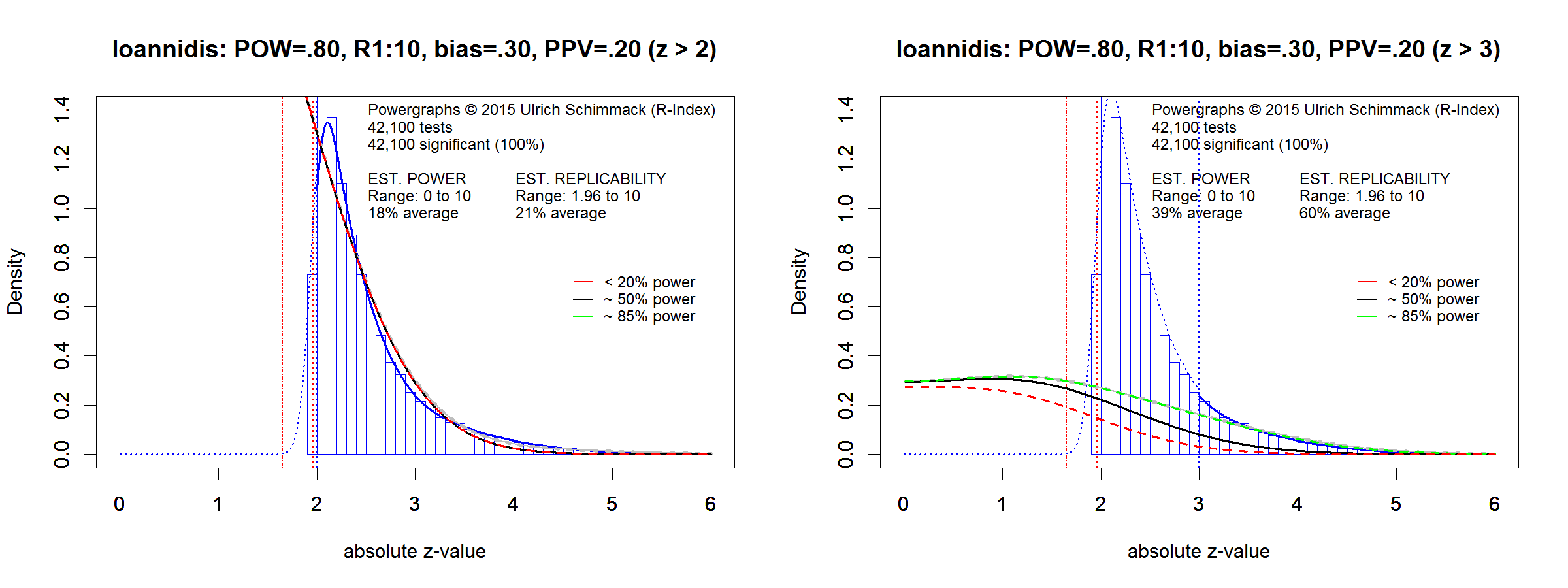A 30% bias implies that for every 100 false hypotheses, there would be 33 (100*[.30*.95+.05]) rather than 5 false positive results (.95*.30+.05)/.95). The effect on false negatives is much smaller (100*[.30*.20 + .80]). Bias was modeled by increasing the number of attempts to produce a significant result so that proportion of true and false hypothesis matched the predicted proportions. Given an assumed 1:10 ratio of true to false hypothesis, the ratio is 335 false hypotheses to 86 true hypotheses. The simulation assumed that researchers tested 100,000 false hypotheses and observed 35000 false positive results and that they tested 10,000 true hypotheses and observed 8,600 true positive results. Bias was simulated by increasing the number of tests to produce the predicted ratio of true and false positive results.

Figure 1 only shows significant results because only significant results would be reported as positive results. Figure 1 shows that a high proportion of z-values are in the range between 1.95 (p = .05) and 3 (p = .001). Powergraphs use z-curve (Schimmack & Brunner, 2016) to estimate the probability that an exact replication study would replicate a significant result. In this simulation, this probability is a mixture of false positives and studies with 80% power. The true average probability is 20%. The z-curve estimate is 21%. Z-curve can also estimate the replicability for other sets of studies. The figure on the right shows replicability for studies that produced an observed z-score greater than 3 (p < .001). The estimate shows an average replicability of 59%. Thus, researchers can increase the chance of replicating published findings by adjusting the criterion value and ignoring significant results with p-values greater than p = .001, even if they were reported as significant with p < .05.

Figure 2 shows the distribution of z-values for Ioannidis’s example of a research program that produces more true than false positives, PPV = .85 (Simulation 1 in Table 4).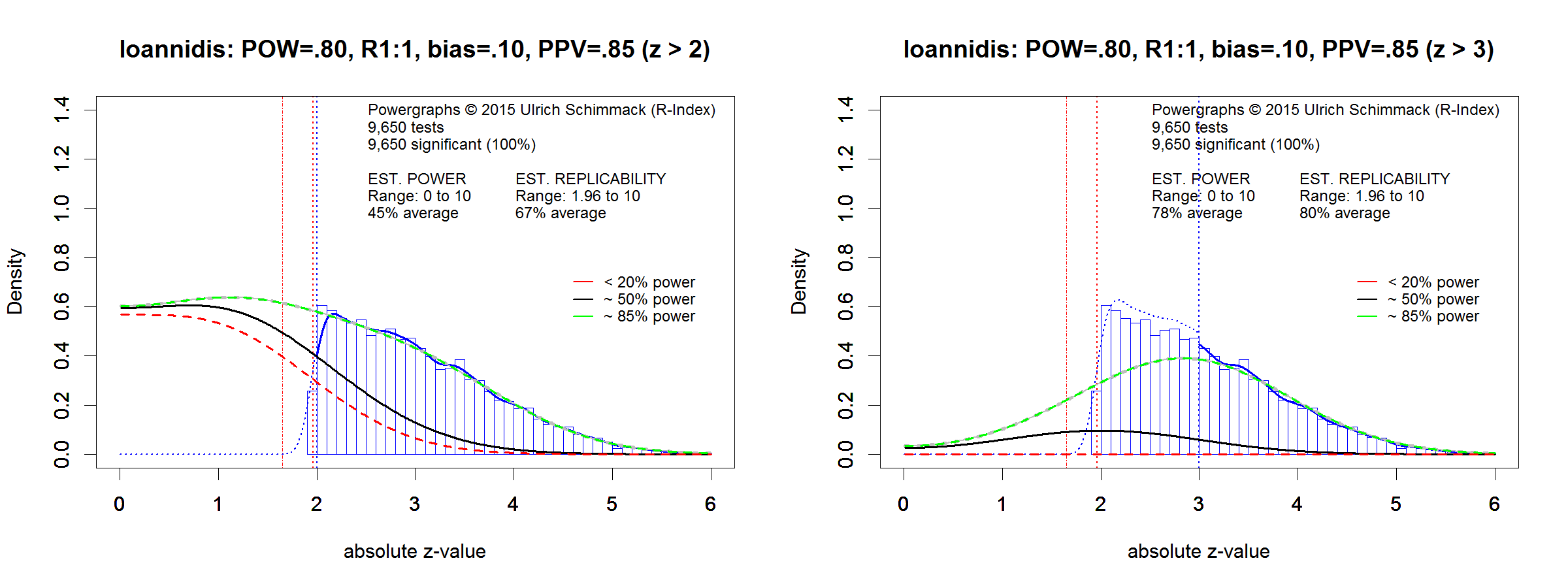Visual inspection of Figure 1 and Figure 2 is sufficient to show that a robust research program produces a dramatically different distribution of z-values. The distribution of z-values in Figure 2 and a replicability estimate of 67% are impossible if most of the published significant results were false.  The maximum value that could be obtained is obtained with a PPV of 50% and 100% power for the true positive results, which yields a replicability estimate of .05*.50 + 1*.50 = 55%. As power is much lower than 100%, the real maximum value is below 50%.

The powergraph on the right shows the replicability estimate for tests that produced a z-value greater than 3 (p < .001). As only a small proportion of false positives are included in this set, z-curve correctly estimates the average power of these studies as 80%. These examples demonstrate that it is possible to test Ioannidis’s claim that most published (significant) results are false empirically. The distribution of test results provides relevant information about the proportion of false positives and power. If actual data are more similar to the distribution in Figure 1, it is possible that most published results are false positives, although it is impossible to distinguish false positives from false negatives with extremely low power. In contrast, if data look more like those in Figure 2, the evidence would contradict Ioannidis’s bold and unsupported claim that most published results are false.

The maximum replicabiltiy that could be obtained with 50% false-positives would require that the true positive studies have 100% power. In this case, replicability would be .50*.05 + .50*1 = 52.5%.  However, 100% power is unrealistic. Figure 3 shows the distribution for a scenario with 90% power and 100% bias and an equal percentage of true and false hypotheses. The true replicabilty for this scenario is .05*.50 + .90 * .50 = 47.5%. z-curve slightly overestimates replicabilty and produced an estimate of 51%.  Even 90% power is unlikely in a real set of data. Thus, replicability estimates above 50% are inconsistent with Ioannidis’s hypothesis that most published positive results are false.  Moreover, the distribution of z-values greater than 3 is also informative. If positive results are a mixture of many false positive results and true positive results with high power, the replicabilty estimate for z-values greater than 3 should be high. In contrast, if this estimate is not much higher than the estimate for all z-values, it suggest that there is a high proportion of studies that produced true positive results with low power.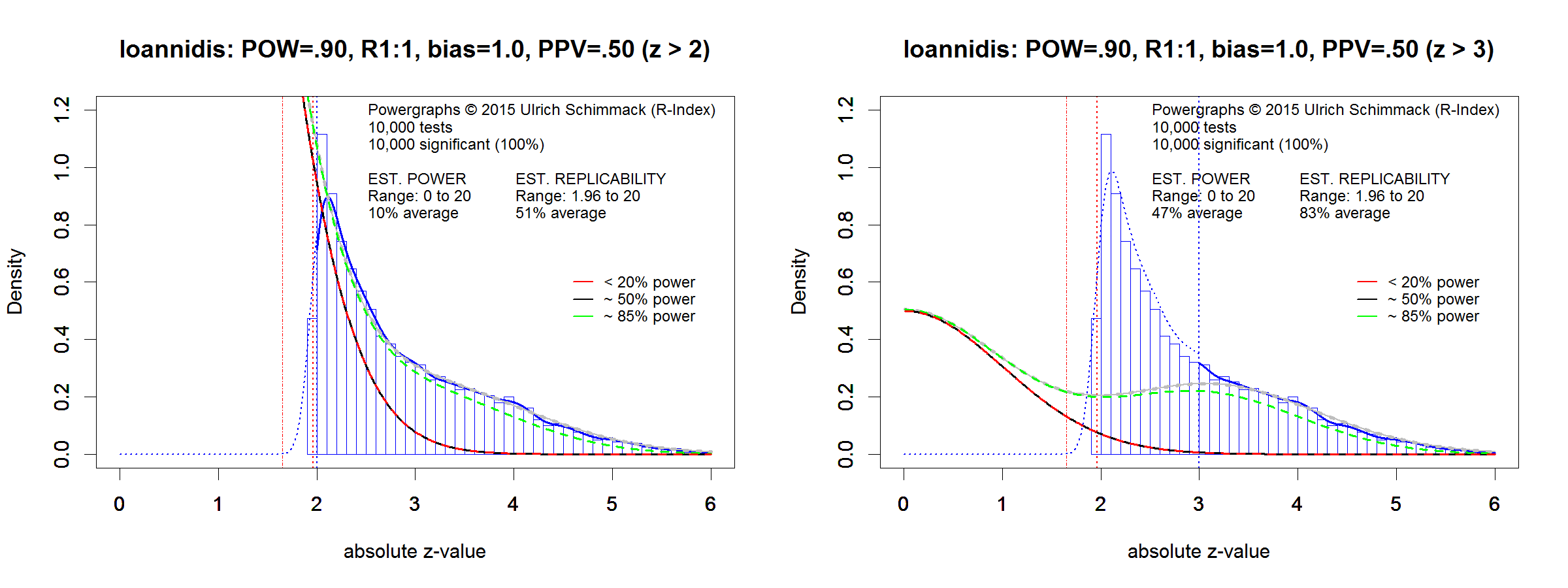Empirical Evidence

I have produced powergraphs and replicability estimates for over 100 psychology journals (2015 Replicabilty Rankings). Not a single journal produced a replicability estimate below 50%. Below are a few selected examples.

The Journal of Experimental Psychology: Learning, Memory and Cognition publishes results from cognitive psychology. In 2015, a replication project (OSC, 2015) demonstrated that 50% of significant results produced a significant result in a replication study. It is unlikely that all non-significant results were false positives. Thus, the results show that Ioannidis’s claim that most published results are false does not apply to results published in this journal.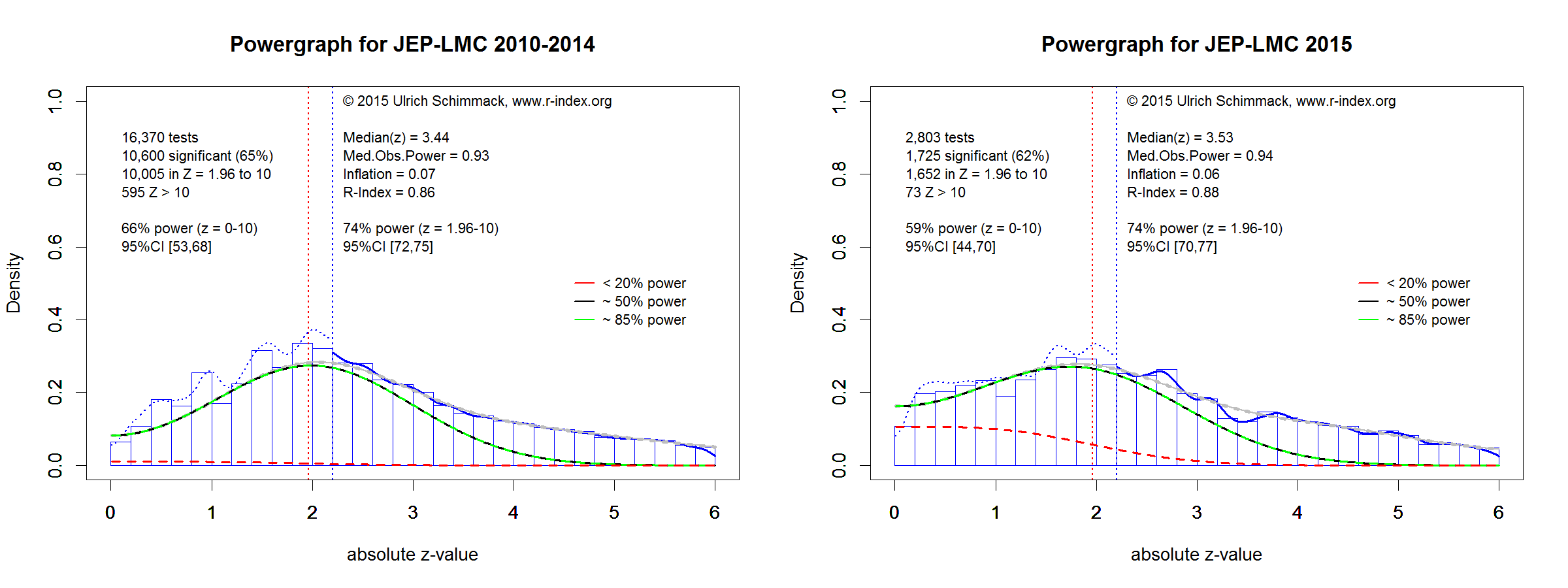The powergraphs further support this conclusion. The graphs look a lot more like Figure 2 than Figure 1 and the replicability estimate is even higher than the one expected from Ioannidis’s simulation with a PPV of 85%.

Another journal that was subjected to replication attempts was Psychological Science. The success rate for Psychological Science was below 50%. However, it is important to keep in mind that a non-significant result in a replication study does not prove that the original result was a false positive. Thus, the PPV could still be greater than 50%.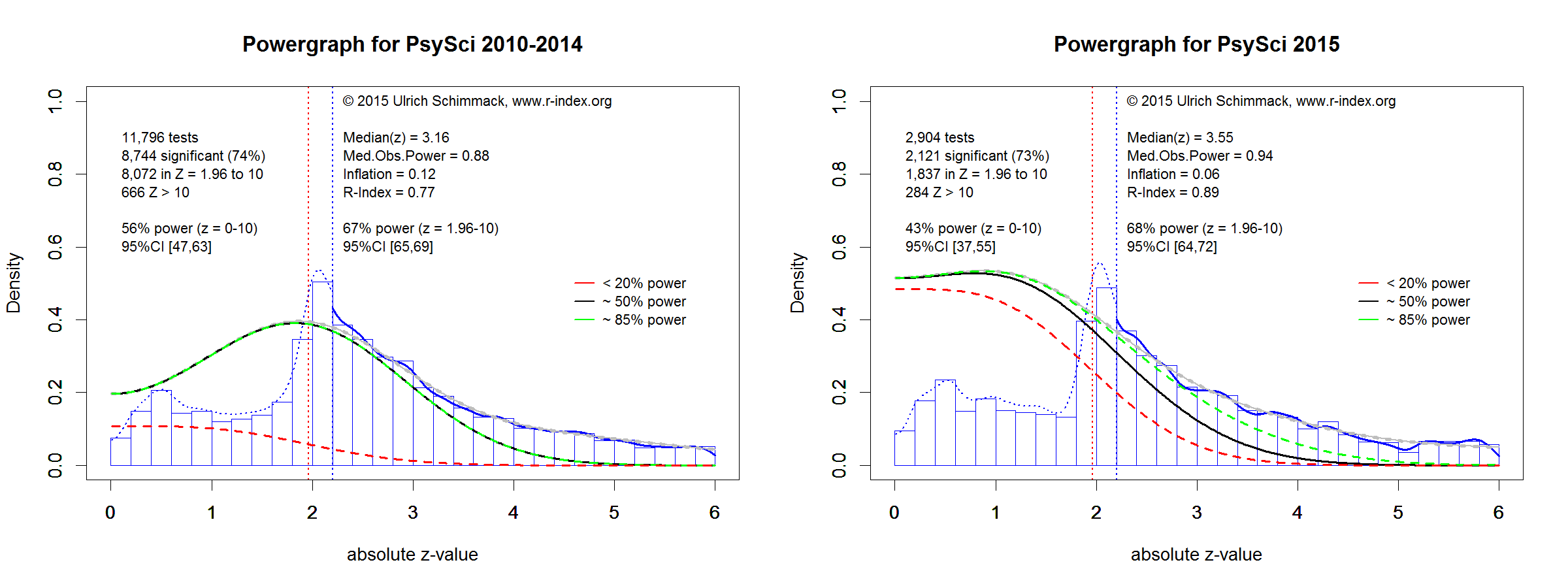The powergraph for Psychological Science shows more z-values in the range between 2 and 3 (p > .001). Nevertheless, the replicability estimate is comparable to the one in Figure 2 which simulated a high PPV of 85%. Closer inspection of the results published in this journal would be required to determine whether a PPV below .50 is plausible.

The third journal that was subjected to a replication attempt was the Journal of Personality and Social Psychology. The journal has three sections, but I focus on the Attitude and Social Cognition section because many replication studies were from this section. The success rate of replication studies was only 25%. However, there is controversy about the reason for this high number of failed replications and once more it is not clear what percentage of failed replications were due to false positive results in the original studies.One problem with the journal rankings is that they are based on automated extraction of all test results. Ioannidis might argue that his claim focused only on test results that tested an original, novel, or an important finding, whereas articles also often report significance tests for other effects. For example, an intervention study may show a strong decrease in depression, when only the interaction with treatment is theoretically relevant.

I am currently working on powergraphs that are limited to theoretically important statistical tests. These results may show lower replicability estimates. Thus, it remains to be seen how consistent Ioannidis’s predictions are for tests of novel and original hypotheses. Powergraphs provide a valuable tool to address this important question.

Moreover, powergraphs can be used to examine whether science is improving. So far, powergraphs of psychology journals have shown no systematic improvement in response to concerns about high false positive rates in published journals. The powergraphs for 2016 will be published soon. Stay tuned.

## 3 thoughts on “Are Most Published Results in Psychology False? An Empirical Study”

1.Eiko Fried says:

Uli, this is great and IMO worth writing up as brief paper.

2.Ruslan says:

It’s not clear from where have you arrived at ‘(7) PTH = alpha/(power + alpha)’. It does not follow from (6).

Also, in Table 1 you construct your ratio such that the numerator and the denominator add up to 100. However, in the last row you change this pattern for some unknown reason.

1.Dr. R says:

Thanks for your comment. As this work is not peer-reviewed, I heavily rely on people like you for feedback.

We can rearrange formula (6) and substitute PFH with (1-PHT) to determine the maximum proportion of true hypotheses to produce over 50% false positive results.

Here is the transformation of (6) to (7)

(7a) = alpha = PTH/(1-PTH) * power

(7b) = alpha*(1-PTH) = PTH * power

(7c) = alpha – PTH*alpha = PTH * power

(7d) = alpha = PTH*alpha + PTH*power

(7e) = alpha = PTH(alpha + power)

(7f) = alpha/(power + alpha) = PTH

The last row in Table 1 had a mistake. It is 33/67